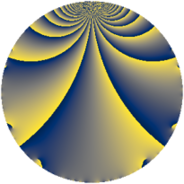# Properties

 Label 495.1.h.aLevel $495$ Weight $1$ Character orbit 495.h Self dual yes Analytic conductor $0.247$ Analytic rank $0$ Dimension $1$ Projective image $D_{2}$ CM/RM discs -11, -55, 5 Inner twists $4$

# Related objects

## Newspace parameters

 Level: $$N$$ $$=$$ $$495 = 3^{2} \cdot 5 \cdot 11$$ Weight: $$k$$ $$=$$ $$1$$ Character orbit: $$[\chi]$$ $$=$$ 495.h (of order $$2$$, degree $$1$$, minimal)

## Newform invariants

 Self dual: yes Analytic conductor: $$0.247037181253$$ Analytic rank: $$0$$ Dimension: $$1$$ Coefficient field: $$\mathbb{Q}$$ Coefficient ring: $$\mathbb{Z}$$ Coefficient ring index: $$1$$ Twist minimal: no (minimal twist has level 55) Projective image: $$D_{2}$$ Projective field: Galois closure of $$\Q(\sqrt{5}, \sqrt{-11})$$ Artin image: $D_4$ Artin field: Galois closure of 4.2.2475.1

## $q$-expansion

 $$f(q)$$ $$=$$ $$q - q^{4} + q^{5}+O(q^{10})$$ q - q^4 + q^5 $$q - q^{4} + q^{5} + q^{11} + q^{16} - q^{20} + q^{25} - 2 q^{31} - q^{44} - q^{49} + q^{55} - 2 q^{59} - q^{64} - 2 q^{71} + q^{80} + 2 q^{89}+O(q^{100})$$ q - q^4 + q^5 + q^11 + q^16 - q^20 + q^25 - 2 * q^31 - q^44 - q^49 + q^55 - 2 * q^59 - q^64 - 2 * q^71 + q^80 + 2 * q^89

## Character values

We give the values of $$\chi$$ on generators for $$\left(\mathbb{Z}/495\mathbb{Z}\right)^\times$$.

 $$n$$ $$46$$ $$56$$ $$397$$ $$\chi(n)$$ $$-1$$ $$1$$ $$-1$$

## Embeddings

For each embedding $$\iota_m$$ of the coefficient field, the values $$\iota_m(a_n)$$ are shown below.

For more information on an embedded modular form you can click on its label.

Label $$\iota_m(\nu)$$ $$a_{2}$$ $$a_{3}$$ $$a_{4}$$ $$a_{5}$$ $$a_{6}$$ $$a_{7}$$ $$a_{8}$$ $$a_{9}$$ $$a_{10}$$
109.1
 0
0 0 −1.00000 1.00000 0 0 0 0 0
 $$n$$: e.g. 2-40 or 990-1000 Significant digits: Format: Complex embeddings Normalized embeddings Satake parameters Satake angles

## Inner twists

Char Parity Ord Mult Type
1.a even 1 1 trivial
5.b even 2 1 RM by $$\Q(\sqrt{5})$$
11.b odd 2 1 CM by $$\Q(\sqrt{-11})$$
55.d odd 2 1 CM by $$\Q(\sqrt{-55})$$

## Twists

By twisting character orbit
Char Parity Ord Mult Type Twist Min Dim
1.a even 1 1 trivial 495.1.h.a 1
3.b odd 2 1 55.1.d.a 1
5.b even 2 1 RM 495.1.h.a 1
5.c odd 4 2 2475.1.b.a 1
11.b odd 2 1 CM 495.1.h.a 1
12.b even 2 1 880.1.i.a 1
15.d odd 2 1 55.1.d.a 1
15.e even 4 2 275.1.c.a 1
21.c even 2 1 2695.1.g.c 1
21.g even 6 2 2695.1.q.b 2
21.h odd 6 2 2695.1.q.c 2
24.f even 2 1 3520.1.i.a 1
24.h odd 2 1 3520.1.i.b 1
33.d even 2 1 55.1.d.a 1
33.f even 10 4 605.1.h.a 4
33.h odd 10 4 605.1.h.a 4
55.d odd 2 1 CM 495.1.h.a 1
55.e even 4 2 2475.1.b.a 1
60.h even 2 1 880.1.i.a 1
105.g even 2 1 2695.1.g.c 1
105.o odd 6 2 2695.1.q.c 2
105.p even 6 2 2695.1.q.b 2
120.i odd 2 1 3520.1.i.b 1
120.m even 2 1 3520.1.i.a 1
132.d odd 2 1 880.1.i.a 1
165.d even 2 1 55.1.d.a 1
165.l odd 4 2 275.1.c.a 1
165.o odd 10 4 605.1.h.a 4
165.r even 10 4 605.1.h.a 4
165.u odd 20 8 3025.1.x.a 4
165.v even 20 8 3025.1.x.a 4
231.h odd 2 1 2695.1.g.c 1
231.k odd 6 2 2695.1.q.b 2
231.l even 6 2 2695.1.q.c 2
264.m even 2 1 3520.1.i.b 1
264.p odd 2 1 3520.1.i.a 1
660.g odd 2 1 880.1.i.a 1
1155.e odd 2 1 2695.1.g.c 1
1155.bh odd 6 2 2695.1.q.b 2
1155.bo even 6 2 2695.1.q.c 2
1320.b odd 2 1 3520.1.i.a 1
1320.u even 2 1 3520.1.i.b 1

By twisted newform orbit
Twist Min Dim Char Parity Ord Mult Type
55.1.d.a 1 3.b odd 2 1
55.1.d.a 1 15.d odd 2 1
55.1.d.a 1 33.d even 2 1
55.1.d.a 1 165.d even 2 1
275.1.c.a 1 15.e even 4 2
275.1.c.a 1 165.l odd 4 2
495.1.h.a 1 1.a even 1 1 trivial
495.1.h.a 1 5.b even 2 1 RM
495.1.h.a 1 11.b odd 2 1 CM
495.1.h.a 1 55.d odd 2 1 CM
605.1.h.a 4 33.f even 10 4
605.1.h.a 4 33.h odd 10 4
605.1.h.a 4 165.o odd 10 4
605.1.h.a 4 165.r even 10 4
880.1.i.a 1 12.b even 2 1
880.1.i.a 1 60.h even 2 1
880.1.i.a 1 132.d odd 2 1
880.1.i.a 1 660.g odd 2 1
2475.1.b.a 1 5.c odd 4 2
2475.1.b.a 1 55.e even 4 2
2695.1.g.c 1 21.c even 2 1
2695.1.g.c 1 105.g even 2 1
2695.1.g.c 1 231.h odd 2 1
2695.1.g.c 1 1155.e odd 2 1
2695.1.q.b 2 21.g even 6 2
2695.1.q.b 2 105.p even 6 2
2695.1.q.b 2 231.k odd 6 2
2695.1.q.b 2 1155.bh odd 6 2
2695.1.q.c 2 21.h odd 6 2
2695.1.q.c 2 105.o odd 6 2
2695.1.q.c 2 231.l even 6 2
2695.1.q.c 2 1155.bo even 6 2
3025.1.x.a 4 165.u odd 20 8
3025.1.x.a 4 165.v even 20 8
3520.1.i.a 1 24.f even 2 1
3520.1.i.a 1 120.m even 2 1
3520.1.i.a 1 264.p odd 2 1
3520.1.i.a 1 1320.b odd 2 1
3520.1.i.b 1 24.h odd 2 1
3520.1.i.b 1 120.i odd 2 1
3520.1.i.b 1 264.m even 2 1
3520.1.i.b 1 1320.u even 2 1

## Hecke kernels

This newform subspace can be constructed as the intersection of the kernels of the following linear operators acting on $$S_{1}^{\mathrm{new}}(495, [\chi])$$:

 $$T_{2}$$ T2 $$T_{89} - 2$$ T89 - 2

## Hecke characteristic polynomials

$p$ $F_p(T)$
$2$ $$T$$
$3$ $$T$$
$5$ $$T - 1$$
$7$ $$T$$
$11$ $$T - 1$$
$13$ $$T$$
$17$ $$T$$
$19$ $$T$$
$23$ $$T$$
$29$ $$T$$
$31$ $$T + 2$$
$37$ $$T$$
$41$ $$T$$
$43$ $$T$$
$47$ $$T$$
$53$ $$T$$
$59$ $$T + 2$$
$61$ $$T$$
$67$ $$T$$
$71$ $$T + 2$$
$73$ $$T$$
$79$ $$T$$
$83$ $$T$$
$89$ $$T - 2$$
$97$ $$T$$